Noise Measurement

The results of the fitted noise values from the threshold scans for all channels are summarized in the plots below. The results are presented in units of DAC counts (left side verical scale) and in SI units (right side vertical scale). The chip numbers are also shown. An average gain of 190 mV/fC was used for the conversion to SI units. The gain measurements are discussed in the following section.

The data before bonding to the silicon are in black.
The data after bonding to the silicon are in red.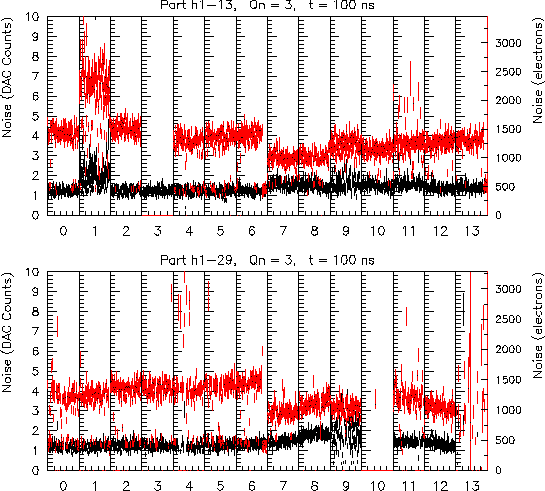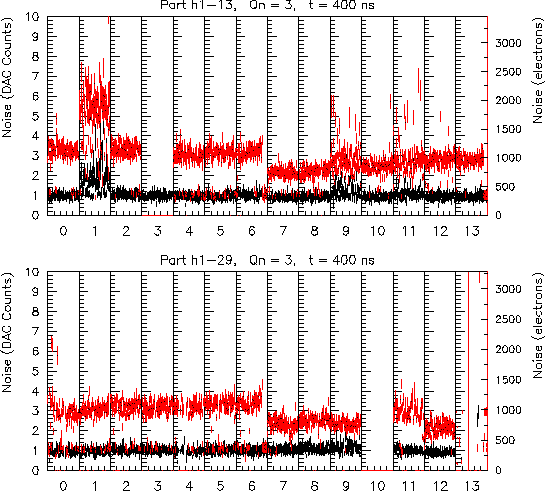The full threshold scan data for the n-side charge injection value of 3 are available in the links below. An example of the presentation format for one chip is given below.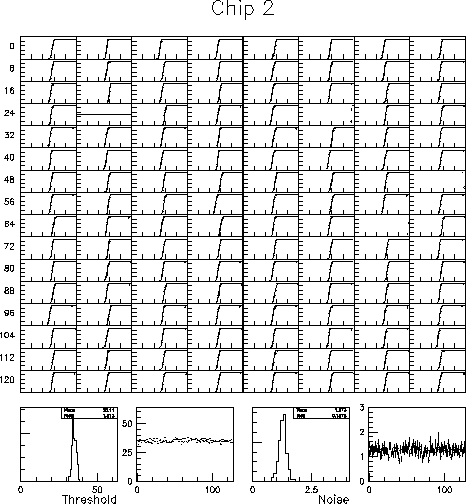Before Bonding to the silicon:
• Threshold scan plots for H1-29, 100ns shaping time, Qn=3.

• Threshold scan plots for H1-13, 100ns shaping time, Qn=3.

• Threshold scan plots for H1-29, 400ns shaping time, Qn=3.

• Threshold scan plots for H1-13, 400ns shaping time, Qn=3.

After Bonding to the silicon:
• Threshold scan plots for H1-29 (D02F-4), 100ns shaping time, Qn=3.

• Threshold scan plots for H1-13 (D02B-8), 100ns shaping time, Qn=3.

• Threshold scan plots for H1-29 (D02F-4), 400ns shaping time, Qn=3.

• Threshold scan plots for H1-13 (D02B-8), 400ns shaping time, Qn=3.

Tables of the average noise for each chip at each charge injection value can be found here .

# Average Noise Values

Qn=2, Qp=61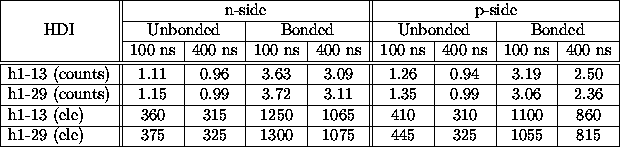Qn=3, Qp=60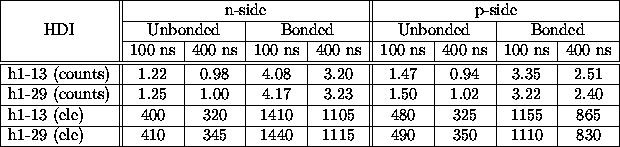Qn=4, Qp=59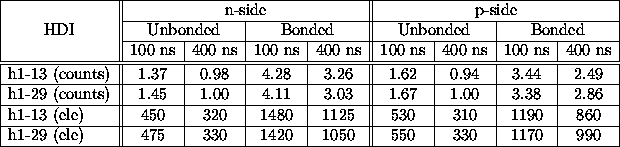The conversion from counts to electrons above assumes an average gain of 200 mV/fC before bonding to the silicon and 190 mV/fC after bonding to the silicon. Chips 1 and 9 from HDI H1-13 and chips 9, 10, and 13 from HDI H1-29 were excluded from the averages due to abnormal behavior.

Some observations: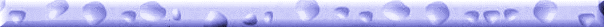Back to contents...
Forward to the next section...